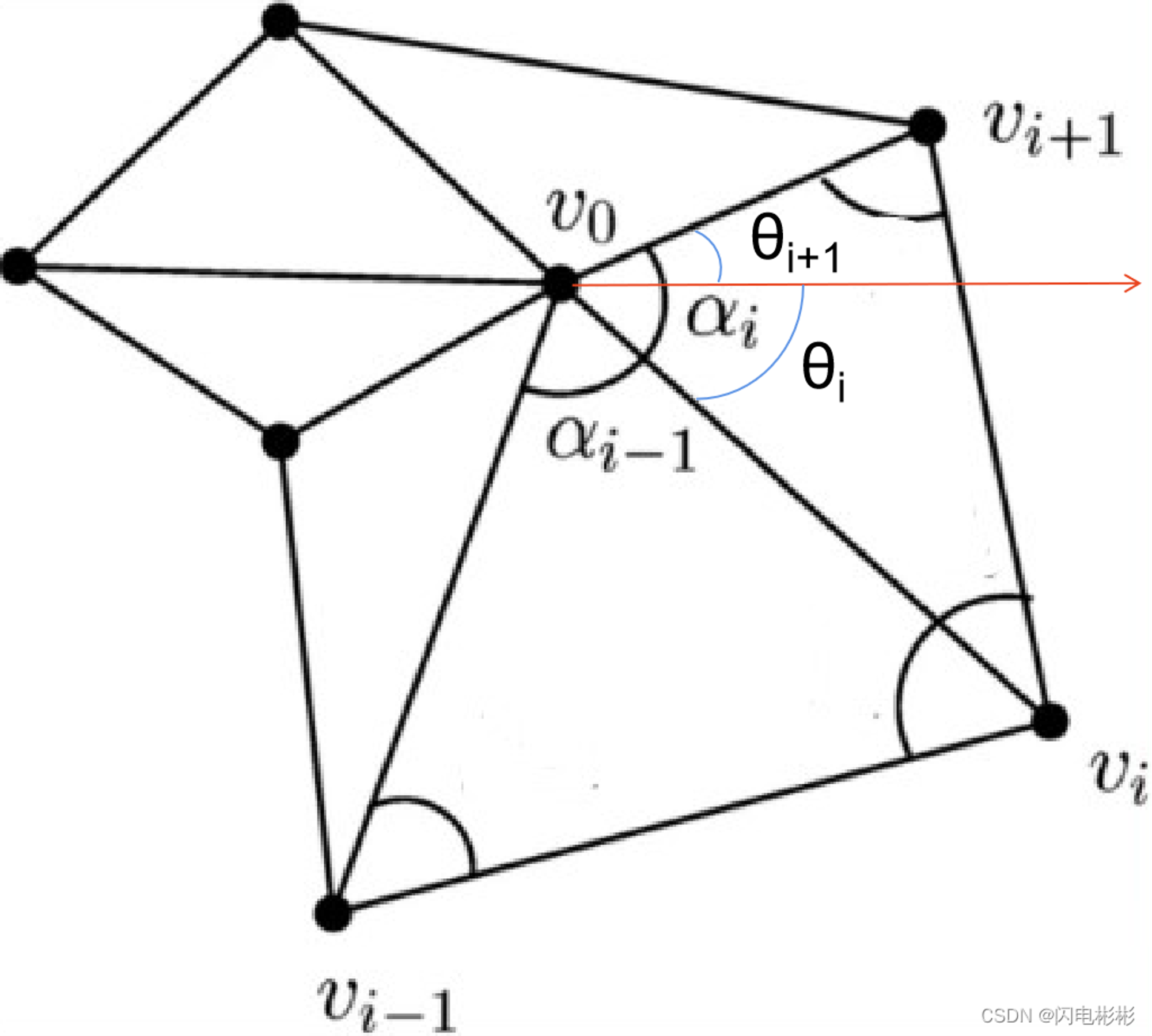V1

2022/10/11阅读：17主题：全栈蓝

# 证明mvc为广义重心坐标引入极坐标定义

tanα=sinα/cosα

sinα+sinβ=2sin[(α+β)/2]cos[(α-β)/2]

sinα-sinβ=2cos[(α+β)/2]sin[(α-β)/2]

cosα+cosβ=2cos[(α+β)/2]cos[(α-β)/2]

cosα-cosβ=-2sin[(α+β)/2]sin[(α-β)/2]V1